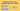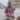# A Helpful Algorithm to Determine "this" value in JavaScript

Updated April 19, 2021Start discussion

Every JavaScript developer, including myself, has been struggling in understanding how `this` keyword works.

I’ve created a universal algorithm to help you determine the value of `this` keyword in any situation.

While I made the algorithm as accessible as possible, I recommend reading it multiple times and understand the related terms.

Also, I’ll show you a step-by-step evaluation of the algorithm for example situations. Finally, try the homework exercises by yourself!

Note: If you don’t understand the algorithm from the first time: that’s expected! Get back to the post later and try again until you crack it.

## 1. this algorithm

The formal definition of `ThisValueOfFunction(func, invocationType)` that returns `this` value a function `func` invoked in a certain way `invocationType`.

ThisValueOfFunction(func, invocationType):

1. If `func` is a regular function, then

1. If `invocationType` is as a constructor, then

1. let `newObject` be the newly constructed object `newObject = new func()`
2. `return newObject`
2. Else if `invocationType` is indirectly, then

1. let `thisArg` be the argument of `func.call(thisArg)` or `func.apply(thisArg)`
2. `return thisArg`
3. Else if `invocationType` is as a method, then

1. let `object` be the object upon which `func` is invoked on `object.func()`
2. `return object`
4. Else if `invocationType` is regular, then

1. If strict mode is enabled, then `return undefined`
2. Else `return globalObject`
2. Else if `func` is an arrow function, then

1. If `func` is defined in the outermost scope, then `return globalObject`
2. Else
1. let `outerFunc` be the outer function of `func`
2. `return ThisValueOfFunction(outerFunc, outerInvocationType)`
3. Else if `func` is a bound function of an `originFunc` function, then

1. let `thisArg` be the argument of `func = originFunc.bind(thisArg)`
2. `return thisArg`
4. Else if `func` is a `constructor()` method inside of a class `SomeClass`, then

1. let `instance` be the instance of the class `instance = new SomeClass()`
2. `return instance`

### 1.1 The terms used in the algorithm

The algorithm uses plenty of JavaScript terms. If you aren’t familiar with something, expand and look at the explanation.

Arrow function

An arrow function is a function defined using the fat arrow syntax `=>`. Example of an arrow function:

`javascript`const sum = (number1, number2) => {  return number1 + number2;}``
Bound function

A bound function is a function created from invoking the method `myFunc.bind(thisArg, arg1, ..., argN)` upon a function. Example of a bound function:

`javascript`function originalFunction() {  // ...}const boundFunction = originalFunction.bind({ prop: 'Value' });``
Regular function

A regular function is a simple JavaScript function defined using `function` keyword or using a shorthand definition on an object. Examples of regular functions:

`javascript`function regularFunction(who) {  return `Hello, \${who}!`;}const object = {  anotherRegularFunction(who) {    return `Good bye, \${who}!`  }};``
constructor()

constructor() is a special method inside of a `class` that initializes the class instance.

`javascript`class SomeClass() {  constructor(prop) {    this.prop = prop;  }}``
Outermost scope

The outermost scope is the top scope that doesn’t have an outer scope.

`javascript`// The outermost scopelet a = 1;function someFunction() {  // someFunction() scope  // Not the outermost scope  let b = 1;}``
Outer function

The outer function contains another function within its scope.

`javascript`// outerFunction() is the outer function of myFunction()function outerFunction() {  function myFunction() {  }}``
Global object

The global object is the object that always exists in the global scope. `window` is the global object in a browser environment, `global` in Node environment.

Invocation

Invocation of a function is just calling the function with some arguments.

`javascript`function sum(number1, number2) {  return number1 + number2;}sum(1, 3);           // Invocationsum.call({}, 3, 4);  // Invocationsum.apply({}, [5, 9]); // Invocationconst obj = {  method() {    return 'Some method';  }};obj.method(); // Invocationclass SomeClass {  constructor(prop) {    this.prop = prop;  } }const instance = new SomeClass('Value'); // Invocation``
Constructor invocation

Constructor invocation happens when a function or class is invoked using `new` keyword.

`javascript`function MyCat(name) {  this.name = name;}const fluffy = new MyCat('Fluffy'); // Constructor invocationclass MyDog {  constructor(name) {    this.name = name;  }}const rex = new MyDog('Rex'); // Constructor invocation``
Indirect invocation

An indirect invocation of happens when a function is called using `func.call(thisArg, ...)` or `func.apply(thisArg, ...)` methods.

`javascript`function sum(number1, number2) {  return number1 + number2;}sum.call({}, 1, 2);  // Indirect invocationsum.apply({}, 3, 5); // Indirect invocation``
Method invocation

Method invocation happens when a function is invoked in a property accessor expression `object.method()`.

`javascript`const object = {  greeting(who) {    return `Hello, \${who}!`  }};object.greeting('World');    // Method invocationobject['greeting']('World'); // Method invocation``
Regular invocation

Regular invocation happens when the sole variable containing the function is used for invocation `func(...)`.

`javascript`function sum(number1, number2) {  return number1 + number2;}sum(1, 4); // Regular invocation``
Strict mode

Strict mode is a special mode imposed upon running JavaScript code having some special restrictions. The strict mode is enabled by adding `'use strict'` directive at the start of the script or the top of the function scope.

## 2. Examples

### Example 1

`javascript`const myFunc = () => {  console.log(this); // logs `window`};myFunc();``

Try the demo.

ThisValueOfFunction(myFunc, “regular”)

`myFunc` is an arrow function: thus matching the point 2 in the algorithm. Also `myFunc` is defined in the outermost scope, matching the point 2.1.

The point 2.1 of the algorithm says `return globalObject`: meaning that `this` value inside `myFunc` is the global object — `window` (in a browser environment).

### Example 2

`javascript`const object = {  method() {    console.log(this); // logs { method() {...} }  } };object.method();``

Try the demo.

ThisValueOfFunction(object.method, “as a method”)

`method()`, while being a property of the `object`, is a regular function. The point 1 of the algorithm is matched.

`object.method()` is a method invocation because of the property accessor usage: thus the point 1.3 is matched.

Then, according to point 1.3, `this` value inside `method()` equals the owning object of the method invocation (`object.method()`) — `object`.

### Example 3

`javascript`function MyCat(name) {  this.name = name;  const getName = () => {    console.log(this); // logs { name: 'Fluffy', getName() {...} }    return this.name;  }  this.getName = getName;}const fluffy = new MyCat('Fluffy');fluffy.getName();``

Try the demo.

ThisValueOfFunction(getName, “as a method”)

`getName()` is an arrow function, thus the point 2 of the algorithm is applied. Then the point 2.2 matches, because `MyCat` is the outer function of `getName()`.

The point 2.2.2 says that `this` value inside `getName()` arrow function equals `this` value of the outer function: `MyCat`.

So, let’s run the algorithm recursively again on `MyCat` function — `ThisValueOfFunction(MyCat, "as a constructor")`.

ThisValueOfFunction(MyCat, “as a constructor”)

`MyCat` is a regular function, thus the point 1 of the algorithm is applied.

Because `MyCat` was invoked as a constructor `new MyCat('Fluffy')`, the point 1.1 is applied. Finally, according to points 1.1.1 and 1.1.2, `this` value inside `MyCat` equals to the constructed object: `fluffy`.

And, returning back to the arrow function’s point 2.2.2, `this` inside of the `getName()` equals `this` of the `MyCat` — which is finally `fluffy`.

## 3. Homework

The best way to understand the algorithm is by trying it yourself. Follow the 3 exercises in determining `this` value.

### Exercise 1

`javascript`const myRegularFunc = function() {  console.log(this); // logs ???};myRegularFunc();``

How would the algorithm determine `this` value inside `myRegularFunc()`? Write the step-by-step evaluation.

### Exercise 2

`javascript`class MyCat {  constructor(name) {    this.name = name;    console.log(this); // logs ???  }}const myCat = new MyCat('Lucy');``

How would the algorithm determine `this` value inside `new MyCat('Lucy')`? Write the step-by-step evaluation.

### Exercise 3

`javascript`const object = {  name: 'Batman',  getName() {    const arrow = () => {      console.log(this); // logs ???      return this.name;    };    return arrow();  };}object.getName();``

How would the algorithm determine `this` value inside `arrow()` function? Write the step-by-step evaluation.

## 4. Summary

In this post, I presented a universal algorithm to determine the value of `this` inside of an invoked function.

While the algorithm might be challenging at first, if you understand the step-by-step examples, you will realize how easy is to apply the algorithm.

Struggle applying the algorithm for a certain situation? Describe your case in a comment below!

## Quality posts into your inbox

I regularly publish posts containing:

• Important JavaScript concepts explained in simple words
• Overview of new JavaScript features
• How to use TypeScript and typing
• Software design and good coding practices